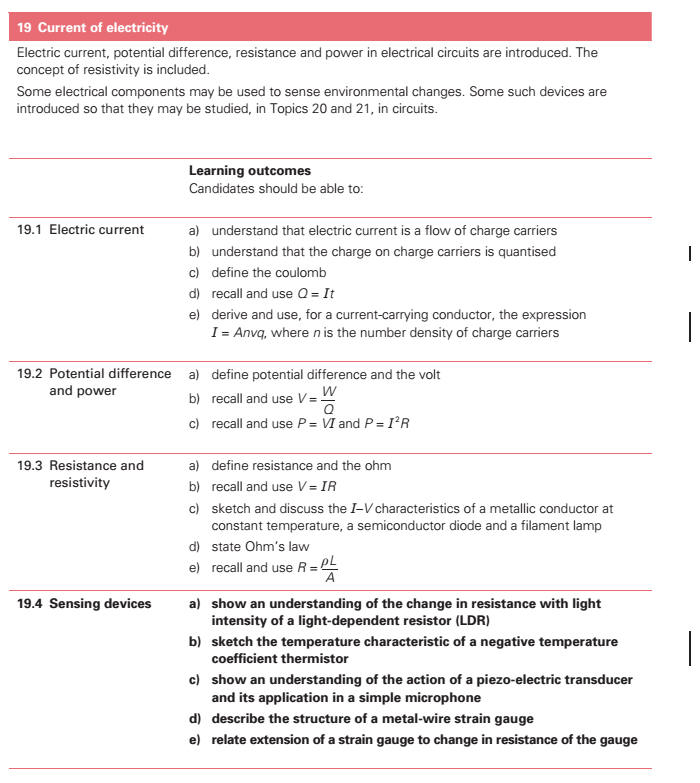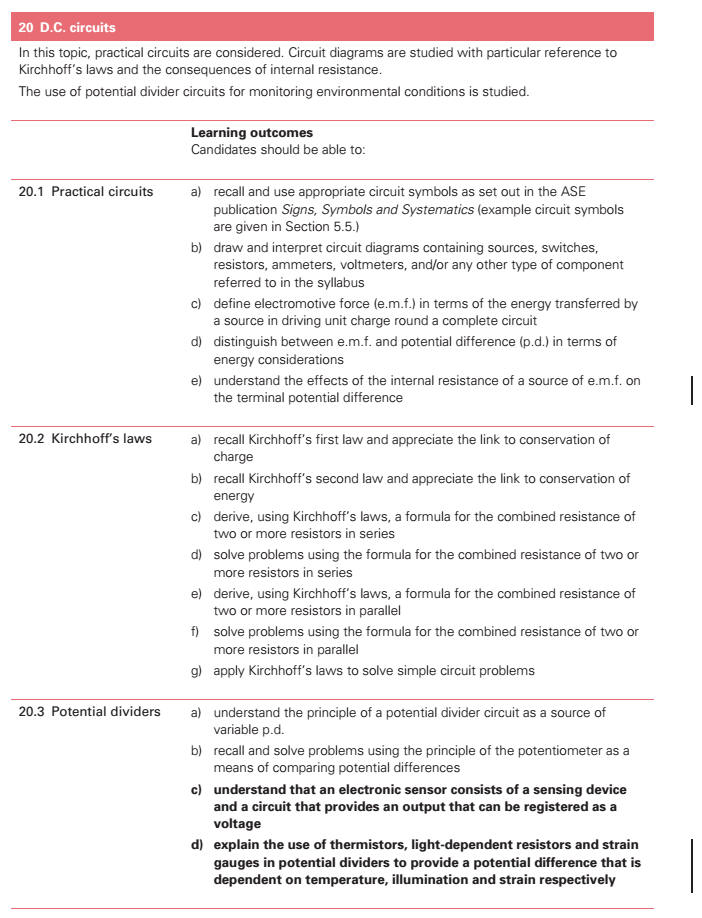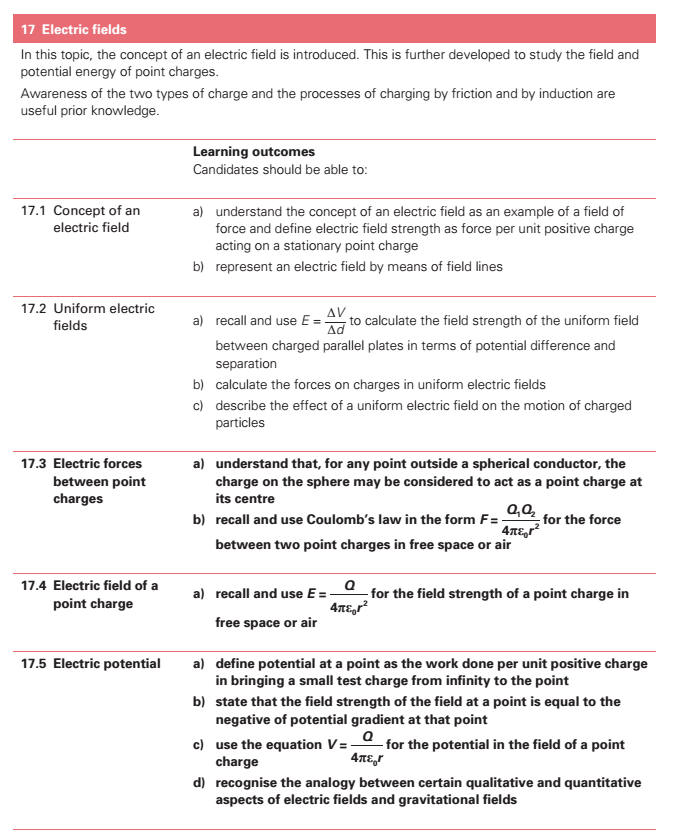19. Current of Electricity (a) show an understanding that electric current is the rate of flow of charged particles. (b) define charge and the coulomb. (c) recall and solve problems using the equation Q = It. (d) define potential difference[][] and the volt. (e) recall and solve problems using V = W/Q. (f) recall and solve problems using P = VI, P = I 2R. (g) define resistance[] and the ohm. (h) recall and solve problems using V = IR. * (i) sketch and explain the I-V characteristics of a metallic conductor at constant temperature, a semiconductor diode and a filament lamp. (j) sketch the temperature[] characteristic of a thermistor[]. (k) state Ohms law. (l) recall and solve problems using R =ρl/A. (m) define e.m.f. in terms of the energy transferred by a source in driving unit charge round a complete circuit. (n) distinguish between e.m.f. and p.d in terms of energy considerations. (o) show an understanding of the effects of the internal resistance[] of a source of e.m.f. on the terminal potential difference[][] and output power[]. 20. D.C. Circuits[] (a) recall and use appropriate circuit symbols as set out in SI Units, Signs, Symbols and Abbreviations (ASE, 1981) and Signs, Symbols and Systematics (ASE, 1995). (b) draw and interpret circuit diagrams containing sources, switches, resistors, ammeters, voltmeters, and/or any other type of component referred to in the syllabus. (c) recall Kirchhoffs first law and appreciate the link to conservation of charge. (d) recall Kirchhoffs second law and appreciate the link to conservation of energy. (e) derive, using Kirchhoffs laws, a formula for the combined resistance[] of two or more resistors in series. (f) solve problems using the formula for the combined resistance[] of two or more resistors in series. (g) derive, using Kirchhoffs laws, a formula for the combined resistance[] of two or more resistors in parallel. (h) solve problems using the formula for the combined resistance[] of two or more resistors in parallel. (i) apply Kirchhoffs laws to solve simple circuit problems. (j) show an understanding of the use of a potential divider[] circuit as a source of variable p.d. * (k) explain the use of thermistors and light-dependent resistors in potential dividers to provide a potential difference[][] which is dependent on temperature[] and illumination respectively. (l) recall and solve problems using the principle of the potentiometer[] as a means of comparing potential differences. 17. Electric Fields[] (a) show an understanding of the concept of an electric field as an example of a field of force and define electric field strength[] as force per unit positive charge. (b) represent an electric field by means of field lines. (c) recall and use E = V/d to calculate the field strength of the uniform field between charged parallel plates in terms of potential difference and separation. (d) calculate the forces on charges in uniform electric fields[]. (e) describe the effect of a uniform electric field on the motion of charged particles.